#### 1600 satellites were sent up by a country for several purposes. The purposes are classified as broadcasting (B), communication (C), surveillance (S), and others (O). A satellite can serve multiple purposes; however a satellite serving either B, or C, or S does not serve O. The following facts are known about the satellites: 1. The numbers of satellites serving B, C, and S (though may be not exclusively) are in the ratio 2: 1:1. 2. The number of satellites serving all three of B, C, and S is 100. 3. The number of satellites exclusively serving C is the same as the number of satellites exclusively serving S. This number is 30% of the number of satellites exclusively serving B. 4. The number of satellites serving O is the same as the number of satellites serving both C and S but not B. Question:If at least 100 of the 1600 satellites were serving O, what can be said about the number of satellites serving S? Option: 1 At most 475   Option: 2 Exactly 475   Option: 3 At least 475   Option: 4 No conclusion is possible based on the given information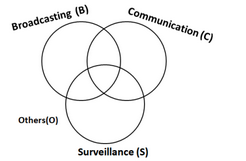Given data:

1. Number of satellites serving B, C, and S (though maybe not exclusively) are in the ratio

2. Number of satellites serving all three of B, C, and S is 100

3. Number of satellites exclusively serving C is the same as the number of satellites exclusively serving S (= 30% of the number of satellites exclusively serving B)

4. Number of satellites serving O is the same as the number of satellites serving both C and S but not B

Since there are satellites serving a single or multiple purposes, Venn Diagram is a good method to solve.

For ease of calculation, let the number of satellites exclusively serving B = 10x.

the number of satellites exclusively serving C and S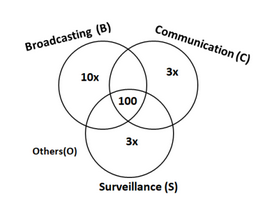Now, let the number of satellites serving others(O) by y.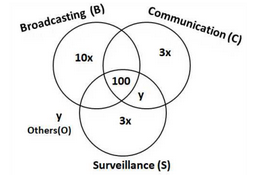Lastly, let the number of satellites serving B, C but not S be z.

Since the numbers of satellites serving B, C, and S (though may be not exclusively) are in the ratio

number of satellites serving B, S but not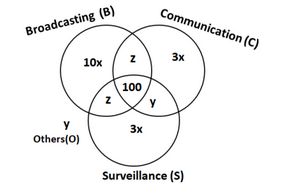It is given that the total number of satellites = 1600:

The numbers of satellites serving B, C, and S (though may be not exclusively) are in the ratio 2:1:1

.......(2)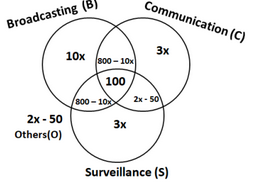Using the boundary condition for x,

Also,

Therefore, we can say that x lies in the range 25 to 80.

Since it is given that at least 100 of the 1600 satellites were serving O.

Number of satellites serving S

At x=75, the number of satellites serving S

At x=80, the number of satellites serving S

Hence, we can say that the number of satellites serving S must be from 425 to 475